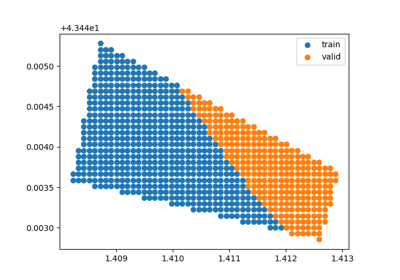# SpatialLeaveAsideOut¶

class `museotoolbox.cross_validation.``SpatialLeaveAsideOut`(distance_matrix, valid_size=0.5, n_repeats=False, random_state=False, verbose=False)[source]

Generate a Cross-Validation using the farthest distance between the training and validation samples.

Parameters
• distance_matrix (numpy.ndarray, shape [n_samples, n_samples]) – Array got from function samplingMethods.getdistance_matrixForDistanceCV(inRaster,inVector)

• valid_size (float, default 0.5.) – The percentage of validaton to keep : from 0 to 1.

• n_repeats (int or bool, optional (default=False)) – If False, n_repeats is 1/valid_size (default : 1/0.5 = 2) If int : will iterate the number of times given in n_repeats.

• random_state (integer or None, optional (default=None)) – If int, random_state is the seed used by the random number generator; If None, the random number generator is created with `time.time()`.

• verbose (integer or False, optional (default=False)) – Controls the verbosity: the higher the value is, the more the messages are detailed.

References

See “Combining ensemble modeling and remote sensing for mapping individual tree species at high spatial resolution” : https://doi.org/10.1016/j.foreco.2013.07.059.

Manage cross-validation methods to generate the duo valid/train samples.

Methods

 `__init__`(distance_matrix[, valid_size, …]) Manage cross-validation methods to generate the duo valid/train samples. `get_n_splits`([X, y, groups]) Returns the number of splitting iterations in the cross-validator. `save_to_vector`(vector, field[, group, …]) Save to vector files each fold from the cross-validation. `split`(X, y[, groups]) Split the vector/array according to y and groups.

## Examples using `museotoolbox.cross_validation.SpatialLeaveAsideOut`¶